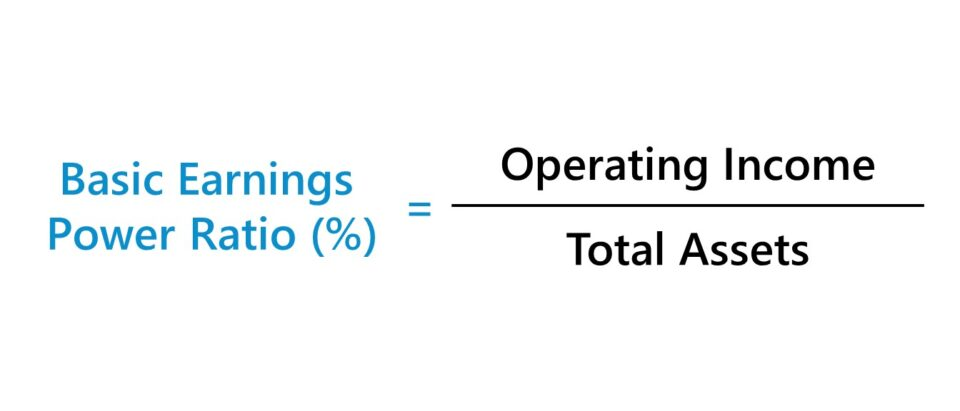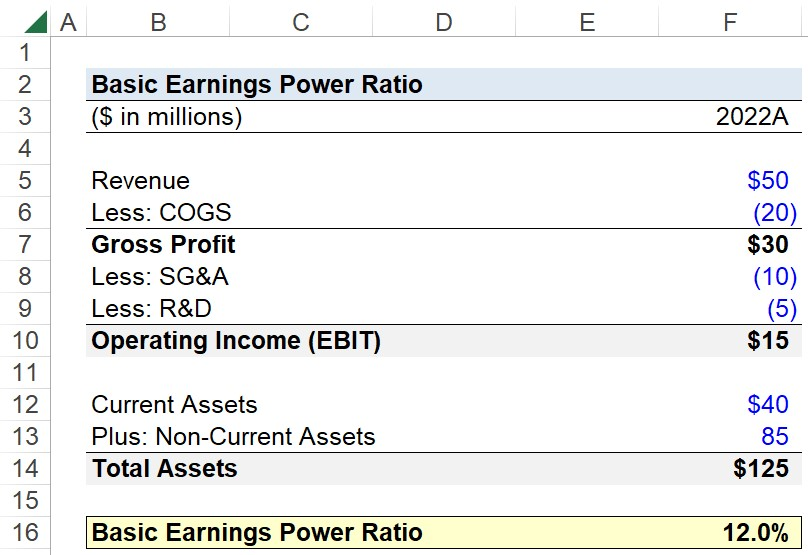# Basic Earnings Power Ratio

Guide to Understanding the Basic Earnings Power Ratio## How to Calculate Basic Earnings Power Ratio (Step-by-Step)

The basic earnings power ratio is a profitability metric that measures how efficiently a company is allocating its resources (i.e. asset base) to generate operating income.

The basic earnings power ratio compares a company’s operating income (EBIT) to the value of its total assets.

• Operating Income: Operating income, or “EBIT”, is the remaining revenue after deducting operating costs, i.e. cost of goods sold (COGS) and operating expenses (SG&A, R&D).
• Total Assets: The total assets of a company are the sum of the value of its current assets (e.g. cash, accounts receivables, inventory) and non-current assets (e.g. PP&E).

The operating income of a company can be found on the income statement, while the value of its total assets is recorded on the balance sheet.

The basic earnings power ratio is one method to understand a company’s earnings capability prior to non-operating items (e.g. interest income, interest expense) and taxes.

Since operating income (EBIT) is the profit metric in the numerator—unlike in the return on assets (ROA) metric—the pre-financial leverage and pre-tax aspect of the basic earnings power ratio makes it more useful for comparisons among industry peers.

• Higher BEP Ratio: The company is more efficient at utilizing its assets to produce income.
• Lower BEP Ratio: The company is less efficient at utilizing its assets to generate income.

## Basic Earnings Power Ratio Formula (BEP)

The formula for calculating the basic earnings power ratio is as follows.

Basic Earnings Power Ratio = Operating Income ÷ Total Assets

Where:

• Operating Income (EBIT) = Gross Profit – Operating Expenses
• Total Assets = Current Assets + Non-Current Assets

Note: The denominator of the ratio, total assets, can be adjusted to include only a company’s operating assets, as well as to remove intangible assets like goodwill. The important rule to follow is to apply the same adjustments to the entire peer group to remain consistent in the calculation.

## Basic Earnings Power Ratio — Excel Model Template

We’ll now move on to a modeling exercise, which you can access by filling out the form below.Submitting ...

## Basic Earnings Power Ratio Calculation Example

Suppose you’re tasked with calculating the basic earnings power ratio of a company given the following assumptions.

• Revenue = \$50 million
• COGS = (\$20 million)
• SG&A = (\$10 million)
• R&D = (\$10 million)
• Current Assets = \$40 million
• Non-Current Assets = \$85 million

The gross profit of the company is \$30 million, which after subtracting its operating expenses (i.e. SG&A and R&D), results in an operating income (EBIT) of \$15 million.

• Gross Profit = \$50 million – \$20 million = \$30 million
• Operating Income (EBIT) = \$30 million – \$10 million – \$5 million = \$15 million

Next, the total assets of our company equal \$125 million, which is simply the sum of its current and non-current assets.

• Total Assets = \$40 million + \$85 million = \$125 million

In conclusion, we’ll calculate our company’s basic earnings power ratio by dividing its operating income (EBIT) by its total assets, which comes out to 12.0%.

• Basic Earnings Power Ratio (%) = \$15 million ÷ \$125 million = 12.0%Step-by-Step Online Course

### Everything You Need To Master Financial Modeling

Enroll in The Premium Package: Learn Financial Statement Modeling, DCF, M&A, LBO and Comps. The same training program used at top investment banks.